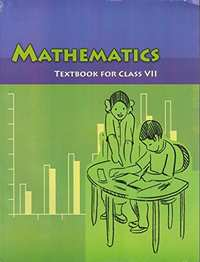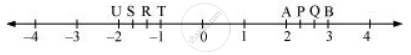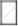# NCERT solutions for Class 7 Maths chapter 9 - Rational Numbers [Latest edition]

#### Chapters## Chapter 9: Rational Numbers

Exercise 9.1Exercise 9.2
Exercise 9.1 [Pages 182 - 184]

### NCERT solutions for Class 7 Maths Chapter 9 Rational Numbers Exercise 9.1 [Pages 182 - 184]

Exercise 9.1 | Q 1.1 | Page 182

List five rational numbers between − 1 and 0

Exercise 9.1 | Q 1.2 | Page 182

List five rational numbers between  − 2 and − 1

−2 and −1

Exercise 9.1 | Q 1.3 | Page 182

List five rational numbers between (-4)/5 and (-2)/3

Exercise 9.1 | Q 1.4 | Page 182

List five rational numbers between 1/2 and 2/3

Exercise 9.1 | Q 2.1 | Page 182

Write four more rational numbers in the following patterns:

(-3)/5, (-6)/10,(-9)/15, (-12)/20, ...

Exercise 9.1 | Q 2.2 | Page 182

Write four more rational numbers in the following patterns:

(-1)/4, (-2)/8,(-3)/12 ....

Exercise 9.1 | Q 2.3 | Page 182

Write four more rational numbers in the following patterns:

(-1)/6, 2/(-12), 3/(-18), 4/(-24)

Exercise 9.1 | Q 2.4 | Page 182

Write four more rational numbers in the following patterns:

(-2)/3, 2/(-3),4/(-6), 6/(-9)...

Exercise 9.1 | Q 3.1 | Page 183

Give four rational numbers equivalent to (-2)/7

Exercise 9.1 | Q 3.2 | Page 183

Give four rational numbers equivalent to 5/(-3)

Exercise 9.1 | Q 3.3 | Page 183

Give four rational numbers equivalent to: 4/9

Exercise 9.1 | Q 4.1 | Page 183

Draw the number line and represent the following rational numbers on it:

3/4

Exercise 9.1 | Q 4.2 | Page 183

Draw the number line and represent the following rational numbers on it:

(-5)/8

Exercise 9.1 | Q 4.3 | Page 183

Draw the number line and represent the following rational numbers on it]

(-7)/4

Exercise 9.1 | Q 4.4 | Page 183

Draw the number line and represent the following rational numbers on it:

7/8

Exercise 9.1 | Q 5 | Page 183

The points P, Q, R, S, T, U, A and B on the number line are such that,

TR = RS = SU and AP = PQ = QB. Name the rational numbers represented by P, Q, R and S.Exercise 9.1 | Q 6.1 | Page 183

Which of the following pairs represent the same rational number?

(-7)/21 and 3/9

Exercise 9.1 | Q 6.2 | Page 183

Which of the following pairs represent the same rational number?

(-16)/20 and 20/(-25)

Exercise 9.1 | Q 6.3 | Page 183

Which of the following pairs represent the same rational number?

(-2)/(-3) and 2/3

Exercise 9.1 | Q 6.4 | Page 183

Which of the following pairs represent the same rational number?

(-3)/5 and (-12)/20

Exercise 9.1 | Q 6.5 | Page 183

Which of the following pairs represent the same rational number?

8/(-5) and (-24)/15

Exercise 9.1 | Q 6.6 | Page 183

Which of the following pairs represent the same rational number?

1/3 and (-1)/9

Exercise 9.1 | Q 6.7 | Page 183

Which of the following pairs represent the same rational number?

(-5)/(-9) and 5/(-9)

Exercise 9.1 | Q 7.1 | Page 183

Rewrite the given rational numbers in the simplest form:

(-8)/6

Exercise 9.1 | Q 7.2 | Page 183

Rewrite the following rational numbers in the simplest form:

25/45

Exercise 9.1 | Q 7.3 | Page 183

Rewrite the following rational numbers in the simplest form:

(-44)/72

Exercise 9.1 | Q 7.4 | Page 183

Rewrite the following rational numbers in the simplest form:

(-8)/10

Exercise 9.1 | Q 8.1 | Page 183

Fill in the boxes with the correct symbol out of >, <, and =

(-5)/72/3

Exercise 9.1 | Q 8.2 | Page 183

Fill in the boxes with the correct symbol out of >, <, and =

(-4)/5(-5)/7

Exercise 9.1 | Q 8.3 | Page 183

Fill in the boxes with the correct symbol out of >, <, and =

(-7)/814/(-16)

Exercise 9.1 | Q 8.4 | Page 183

Fill in the boxes with the correct symbol out of >, <, and =

(-8)/5(-7)/4

Exercise 9.1 | Q 8.5 | Page 183

Fill in the boxes with the correct symbol out of >, <, and =

1/(-3)(-1)/4

Exercise 9.1 | Q 8.6 | Page 183

Fill in the boxes with the correct symbol out of >, <, and =

5/(-11)(-5)/11

Exercise 9.1 | Q 8.7 | Page 183

Fill in the boxes with the correct symbol out of >, <, and =

0(-7)/6

Exercise 9.1 | Q 9.1 | Page 184

Which is greater in the following?

2/3,5/2

Exercise 9.1 | Q 9.2 | Page 184

Which is greater in the following?

(-5)/6, (-4)/3

Exercise 9.1 | Q 9.3 | Page 184

Which is greater in the following?

(-3)/4,2/(-3)

Exercise 9.1 | Q 9.4 | Page 184

Which is greater in the following?

(-1)/4, 1/4

Exercise 9.1 | Q 9.5 | Page 184

Which is greater in the following?

-3 2/7, -3 4/5

Exercise 9.1 | Q 10.1 | Page 184

Write the given rational numbers in ascending order:

(-3)/5, (-2)/5, (-1)/5

Exercise 9.1 | Q 10.2 | Page 184

Write the given rational numbers in ascending order:

(-1)/3,(-2)/9,(-4)/3

Exercise 9.1 | Q 10.3 | Page 184

Write the given rational numbers in ascending order:

(-3)/7, (-3)/2, (-3)/4

Exercise 9.2 [Page 190]

### NCERT solutions for Class 7 Maths Chapter 9 Rational Numbers Exercise 9.2 [Page 190]

Exercise 9.2 | Q 1.1 | Page 190

Find the sum 4/5 + ((-11)/4)

Exercise 9.2 | Q 1.2 | Page 190

Find the sum 5/3 + 3/5

Exercise 9.2 | Q 1.3 | Page 190

Find the sum (-9)/10 + 22/15

Exercise 9.2 | Q 1.4 | Page 190

Find the sum: (-3)/(-11) + 5/9

Exercise 9.2 | Q 1.5 | Page 190

Find the sum: (-8)/19 + (-2)/57

Exercise 9.2 | Q 1.6 | Page 190

Find the sum: (-2)/3 + 0

Exercise 9.2 | Q 1.7 | Page 190

Find the sum: -2 1/3 + 4 3/5

Exercise 9.2 | Q 2.1 | Page 190

Find 7/24 - 17/36

Exercise 9.2 | Q 2.2 | Page 190

Find 5/63 - ((-6)/21)

Exercise 9.2 | Q 2.3 | Page 190

Find (-6)/13 - ((-17)/15)

Exercise 9.2 | Q 2.4 | Page 190

Find (-3)/8 - 7/11

Exercise 9.2 | Q 2.5 | Page 190

Find -2 1/9 - 6

Exercise 9.2 | Q 3.1 | Page 190

Find the product: 9/2 xx ((-7)/4)

Exercise 9.2 | Q 3.2 | Page 190

Find the product: 3/10 xx (-9)

Exercise 9.2 | Q 3.3 | Page 190

Find the product: (-6)/9 xx 9/11

Exercise 9.2 | Q 3.4 | Page 190

Find the product: 3/7 xx ((-2)/5)

Exercise 9.2 | Q 3.5 | Page 190

Find the product: 3/11 xx 2/5

Exercise 9.2 | Q 3.6 | Page 190

Find the product: 3/(-5) xx (-5)/3

Exercise 9.2 | Q 4.1 | Page 190

Find the value of (-4) ÷ 2/3

Exercise 9.2 | Q 4.2 | Page 190

Find the value of: (-3)/5 ÷ 2

Exercise 9.2 | Q 4.3 | Page 190

Find the value of: (-4)/5 ÷ (-3)

Exercise 9.2 | Q 4.4 | Page 190

Find the value of: (-1)/8 ÷ 3/4

Exercise 9.2 | Q 4.5 | Page 190

Find the value of: (-2)/13 ÷ 1/7

Exercise 9.2 | Q 4.6 | Page 190

Find the value of: (-7)/12 ÷ ((-2)/13)

Exercise 9.2 | Q 4.7 | Page 190

Find the value of: 3/13 ÷ ((-4)/65)

## Chapter 9: Rational Numbers

Exercise 9.1Exercise 9.2## NCERT solutions for Class 7 Maths chapter 9 - Rational Numbers

NCERT solutions for Class 7 Maths chapter 9 (Rational Numbers) include all questions with solution and detail explanation. This will clear students doubts about any question and improve application skills while preparing for board exams. The detailed, step-by-step solutions will help you understand the concepts better and clear your confusions, if any. Shaalaa.com has the CBSE Class 7 Maths solutions in a manner that help students grasp basic concepts better and faster.

Further, we at Shaalaa.com provide such solutions so that students can prepare for written exams. NCERT textbook solutions can be a core help for self-study and acts as a perfect self-help guidance for students.

Concepts covered in Class 7 Maths chapter 9 Rational Numbers are Concept of Rational Numbers, Positive and Negative Rational Numbers, Rational Numbers on a Number Line, Rational Numbers in Standard Form, Comparison of Rational Numbers, Rational Numbers Between Two Rational Numbers, Addition of Rational Number, Subtraction of Rational Number, Multiplication of Rational Numbers, Division of Rational Numbers, Equivalent Rational Number.

Using NCERT Class 7 solutions Rational Numbers exercise by students are an easy way to prepare for the exams, as they involve solutions arranged chapter-wise also page wise. The questions involved in NCERT Solutions are important questions that can be asked in the final exam. Maximum students of CBSE Class 7 prefer NCERT Textbook Solutions to score more in exam.

Get the free view of chapter 9 Rational Numbers Class 7 extra questions for Class 7 Maths and can use Shaalaa.com to keep it handy for your exam preparation